# Standard Algorithm

Page 4 of 5

Let's go!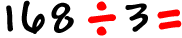Set it up: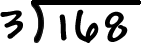Do the
DMS loopDivision, multiplication, subtraction!

 Division: How many times does 3 go into 1?  Hey, 3 doesn't go into 1...  3 is bigger than 1! No problem...  We'll just use the next number too!How many times 3 will go into 16?  Three goes into 16 five times with a little extra.  Put that 5 right above the 6: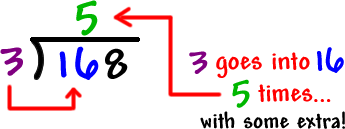Multiplication: Multiply the 5 and the 3 and put the answer right under the 16: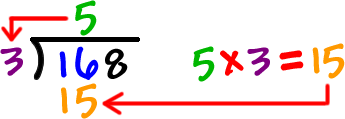Subtraction: Do the subtraction...That's 16 - 15 = 1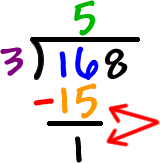First DMS loop done!  Almost there!!

 Set it up:  Drag the 8 down. That makes it a 18...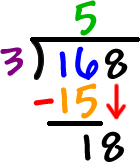Now, let's go back into our DMS loop using the 18!

 Division: How many times 3 will go into 18?  Three goes into 18 cleanly... six times.  Put that 6 right above the 8: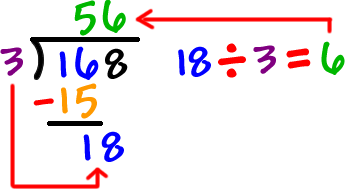Multiplication: Multiply the 6 and the 3 and put the answer right under the 18: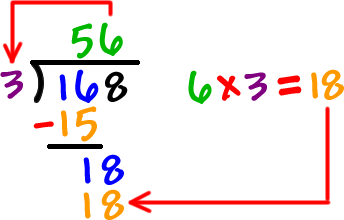Subtraction: That's 18 - 18 = 0 Yeah, baby!  Done! The answer is 56.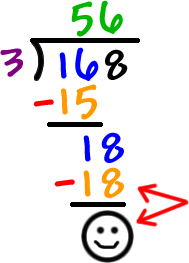Check it!!  56 x 3 should = 168!

OK, ready to work some along with me?  Go to the next page to get started!